Tutorial 12: Waveshaping
Using a stored wavetable
In Tutorial 3 we used 512 samples stored in a buffer~ as a wavetable to be read by the cycle~ object. The name of the buffer~ object is typed in as an argument to the cycle~ object, causing cycle~ to use samples from the buffer~ as its waveform, instead of its default cosine wave. The frequency value received in the left inlet of the cycle~ determines how many times per second it will read through those 512 samples, and thus determines the fundamental frequency of the tone it plays.
Just to serve as a reminder, an example of that type of wavetable synthesis is included in the lower right corner of this tutorial patch.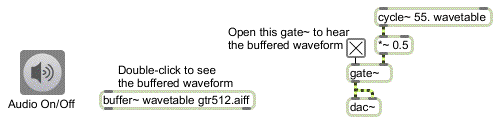The cycle~ object reads repeatedly through the 512 samples stored in the buffer~
• Double-click on the buffer~ object to see its contents. The file gtr512.aiff contains one cycle of a recorded electric guitar note. Click on the ezdac~ speaker icon to turn audio on. Click on the toggle to open the gate~, allowing the output of cycle~ to reach the dac~. Click on the toggle again to close the gate~.
This type of synthesis allows you to use any waveform for cycle~, but the timbre is static and somewhat lifeless because the waveform is unchanging. This tutorial presents a new way to obtain dynamically changing timbres, using a technique known as waveshaping.
Table lookup: lookup~
In waveshaping synthesis an audio signal -- most commonly a sine wave -- is used to access a lookup table containing some shaping function (also commonly called a transfer function). Each sample value of the input signal is used as an index to look up a value stored in a table (an array of numbers). Because a lookup table may contain any values in any order, it is useful for mapping a linear range of values (such as the signal range -1 to 1) to a nonlinear function (whatever is stored in the lookup table). The Max object table is an example of a lookup table; the number received as input (commonly in the range 0 to 127) is used to access whatever values are stored in the table.
The MSP object lookup~ allows you to use samples stored in a buffer~ as a lookup table which can be accessed by a signal in the range -1 to 1. By default, lookup~ uses the first 512 samples in a buffer~, but you can type in arguments to specify any excerpt of the buffer~ object's contents for use as a lookup table. If 512 samples are used, input values ranging from -1 to 0 are mapped to the first 256 samples, and input values from 0 to 1 are mapped to the next 256 samples; lookup~ interpolates between two stored values as necessary.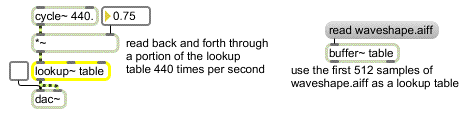Sine wave used to read back and forth through an excerpt of the buffer~
The most commonly used input signal for indexing the lookup table is a sine wave -- it's a reasonable choice because it reads smoothly back and forth through the table -- but any audio signal can be used as input to lookup~.
The important thing to observe about waveshaping synthesis is this: changing the amplitude of the input signal changes the amount of the lookup table that gets used. If the range of the input signal is from -1 to 1, the entire lookup table is used. However, if the range of the input signal is from -0.33 to 0.33, only the middle third of the table is used. As a general rule, the timbre of the output signal becomes brighter (contains more high frequencies) as the amplitude of the input signal increases.
It's also worth noting that the amplitude of the input signal has no direct effect on the amplitude of the output signal; the output amplitude depends entirely on the values being indexed in the lookup table.
Varying timbre with waveshaping
The waveshaping part of the tutorial patch is in the lower left portion of the Patcher window. It's very similar to the example shown above.

The lookup table consists of the 512 samples in the buffer~, and it is read by a cosine wave from a cycle~ object.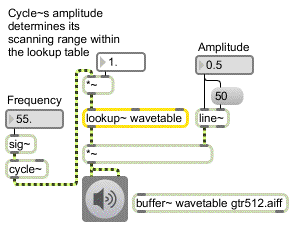Lookup table used for waveshaping
The upper portion of the Patcher window contains three different ways to vary the amplitude of the cosine wave, which will vary the timbre.
• With the audio still on, choose ‘Set range by hand’ from the pop-up umenu. This opens the first signal inlet of the selector~, so you can alter the amplitude of the cycle~ by dragging in the number box marked ‘By hand’. Change the value in the number box to hear different timbres.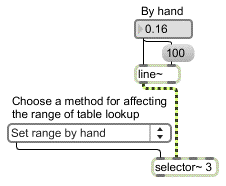Set the amplitude of the input signal to change the timbre of the output
To make the timbre change over the course of the note, you can use a control function envelope to vary the amplitude of the cycle~ automatically over time.
• Choose ‘Control range by envelope’ from the umenu. Set a note duration by typing a value into the number box marked ‘Duration’ (such as 1000 ms), then click on the button to play a note. Experiment with different durations and envelopes.
You can also modulate the amplitude of the input wave with another signal. An extremely slow modulating frequency (such as 0.1 Hz) will change the timbre very gradually. A faster sub-audio modulating frequency (such as 8 Hz) will create a unique sort of ‘timbre tremolo’. Modulating the input wave at an audio rate creates sum and difference frequencies (as you have seen in Tutorial 9) which may interfere in various ways depending on the modulation rate.
• Choose ‘Modulate range by wave’ from the umenu. Set the modulation rate to 0.1 Hz and set the modulation depth to 0.9.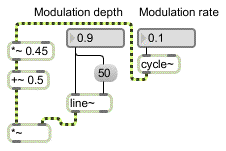Very slow modulation of the input wave's amplitude creates a gradual timbre change
Notice that the amplitude of the cycle~ is multiplied by 0.45 and offset by 0.5. That makes it range from 0.05 to 0.95. (If it went completely to 0 the amplitude of the wave it's modulating would be 0 and the sound would stop.) The ‘Modulation depth’ number box goes from 0 to 1, but it's actually scaling the cycle~ within that range from 0.05 to 0.95.
• Experiment with other values for the depth and rate of modulation.
If you're designing an instrument for musical purposes, you might use some combination of these three ways to vary the timbre, and you almost certainly would have an independent amplitude envelope to scale the amplitude of the output sound. (Remember that the amplitude of the signal coming out of lookup~ depends on the sample values being read, and is not directly affected by the amplitude of the signal coming into it.)
Summary
Waveshaping is the nonlinear distortion of a signal to create a new timbre. The sample values of the original signal are used to address a lookup table, and the corresponding value from the lookup table is sent out. The lookup~ object treats samples from a buffer~ as such a lookup table, and uses the input range -1 to 1 to address those samples. A sine wave is commonly used as the input signal for waveshaping synthesis. The amplitude of the input signal determines how much of the lookup table gets used. As the amplitude of the input signal increases, more of the table gets used, and consequently more frequencies are generally introduced into the output. Thus, you can change the timbre of a waveshaped signal dynamically by continuously altering the amplitude of the input signal, using a control function or a modulating signal.